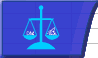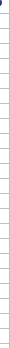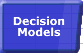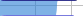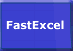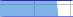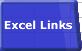# Optimising LookupsImportance of Speed Faster Calculations Microsoft's Advice Bottlenecks/size Lookups Dynamic Ranges Totalling Generating Workbooks Multi-Level Calculations Array Formulae SUMIF Example Worksheet LinksLookup Options Speeding Up Lookups Missing Values Multiple Cells Two-Dimensional Lookup Three-Dimensional Lookup

## Lookup Options

Lookups are often the most significant factor in calculation times, and fortunately there are many ways of improving lookup calculation time.

With FastExcel Version 2 you can now use the AVLOOKUP function, which is faster than VLOOKUP and INDEX/MATCH in many circumstances.

#### Make sure you have understood the options in MATCH, VLOOKUP and HLOOKUP.

MATCH(lookup_value, lookup_array, match_type)

• Match_type=1 returns the largest match less than or equal to lookup value if lookup array is sorted ascending. This is the default
• Match_type=0 requests an exact match
• Match_type=-1 returns the smallest match greater than or equal to lookup value if lookup array is sorted descending

VLOOKUP(lookup_value, table_array, colnum, range_lookup)

• Range_lookup=TRUE returns the largest match less than or equal to lookup value. This is the default option. Table array MUST be sorted ascending.
• Range_lookup=FALSE requests an exact match. Table array does not need to be sorted.
 Avoid using exact match lookup if possible. If you are doing lookup’s using the exact match option the calculation time for the function is proportional to the number of cells scanned before a match is found. For lookups over large ranges this time can be very significant. Lookup time using the approximate match options of VLOOKUP, HLOOKUP, MATCH on sorted data is fast and not significantly increased by the length of the range you are looking up. (Characteristics are the same as binary search).

#### VLOOKUP & MATCH with multiple matches.

If the table you are looking up contains more than one row with a value which matches your lookup value, which row gets found?

• If the table is not sorted the first matching row found is returned.
• If the table is sorted ascending then the last matching row is returned.

#### VLOOKUP versus INDEX and MATCH or OFFSET.

 I recommend using INDEX and MATCH. VLOOKUP is slightly faster (approx. 5%), simpler and uses less memory than a combination of MATCH and INDEX or OFFSET. However the additional flexibility offered by MATCH and INDEX often allows you to make significant timesaving compared to VLOOKUP. INDEX is very fast and from Excel 97 onwards is a non-volatile function (speeds up recalculation). OFFSET is also very fast, but it’s a volatile function.

#### Converting VLOOKUP to INDEX and MATCH.

These statements return the same answer:

VLOOKUP(A1, Data!\$A\$2:\$F\$1000,3,False)
INDEX(Data!\$A\$2:\$F\$1000,MATCH(A1,\$A\$1:\$A\$1000,0),3)

### Speeding up Lookup’s

Because exact match lookups are so slow it’s worth looking for ways of speeding things up:

#### Use FastExcel's AVLOOKUP function.

AVLOOKUP is significantly faster than VLOOKUP in many circumstances.

#### Use One Worksheet.

 If speed is critical keep Lookups and Data on the same sheet. Keep exact match lookups on the same worksheet as the data they are looking up: It’s significantly faster.

#### Use Excel 2000 or later:

 Upgrade to Excel 2000. Excel 2000 is significantly faster than Excel 97 for exact matches. But make sure you have installed the SR1 Service Release which fixes a problem with Lookups into closed workbooks (see MSKB Q248173).

#### SORT the Data Whenever Possible.

 SORT your data and use approximate Match. Whenever possible SORT the data first, (SORT is very fast) and use approximate match.

#### Minimise the Range of Cells you are Looking Up.

 The smaller the Range the better. When doing exact match lookups restrict the range of cells to be scanned to a minimum. Use Dynamic Range Names rather than referring to a very large number of rows or columns. Sometimes you can pre-calculate a lower and upper range limit for the lookup.

#### Sorted Data with Missing Values.

 Two approximate Lookups are usually faster than one exact Lookup. If you can sort your data but still cannot use approximate match because you can’t be sure that the value you are looking up exists in the lookup range, then try this: IF(lookup_val=Index(lookup_array,MATCH(lookup_val,lookup_list),1) ,Index(lookup_array,MATCH(lookup_val,lookup_array), colnum),“notexist”) This does an approximate lookup on the lookup list, and if the lookup value = the answer in the lookup column you have found an exact match, so redo the approximate lookup on the column you want, otherwise it’s a missing value. Note that this assumes you never lookup a value smaller than the smallest value in the list, so you may need to add a dummy very small entry into the list. Two approximate matches are significantly faster than one exact match for a lookup over a large number of rows (breakeven point is about 10-20 rows). FastExcel’s AVLOOKUP function allows you to handle the missing value problem very efficiently.

#### Unsorted Data with Missing Values.

 Store the result of an exact MATCH and reuse it. If you have to use exact match lookup on unsorted data and you can’t be sure that the lookup value exists you often have to handle the #N/A that gets returned if no match is found. The simplest and slowest way is to use an IF function containing two lookups: IF(ISNA(VLOOKUP(lookupval,table,2,FALSE)),0, VLOOKUP(lookupval,table,2,FALSE)) You can avoid the double exact lookup if you use exact MATCH once, store the result in a cell, and then test the result before doing an INDEX: In A1 =MATCH(lookupvalue,lookuparray,0) In B1=IF(ISNA(A1),0,INDEX(table,A1,colnum)) If you cannot use two cells then use COUNTIF, on average it is faster than an exact match lookup: IF (COUNTIF(lookuparray,lookupvalue)=0, 0, VLOOKUP(lookupval, table, 2 FALSE)) FastExcel’s AVLOOKUP function allows you to handle the missing value problem very efficiently

#### Exact Match Lookups returning values from Multiple Columns.

 You can often reuse a stored exact MATCH many times. If you are doing exact lookups on multiple columns you can save a lot of time using one MATCH and many INDEX statements rather than many VLOOKUPs. Add an extra column for the MATCH to store the result (stored_row). For each column use: INDEX(Lookup_Range,stored_row,column_number) Alternatively you can use VLOOKUP in an array formula: this example returns the value from the 2nd and 4th column in the lookup range. {VLOOKUP(lookupvalue,Lookup_Range,{4,2},FALSE)}

#### Looking Up a Set of Contiguous Rows or Columns.

 Y ou can also return many cells from one Lookup operation. If you want to lookup a number of contiguous columns then you can use INDEX in an array formula to return multiple columns at once (use 0 as the column number). You can also use INDEX to return multiple rows at once. {INDEX(\$A\$1:\$J\$1000,stored_row,0)} This returns columns A to J in the stored row created by a previous MATCH

#### Looking Up a Rectangular Block of Cells.

You can use MATCH and OFFSET to return a rectangular block of cells as a range.

#### Two-Dimensional Lookup

 Multi-dimensional lookup can also be done efficiently. Two-dimensional table lookup using separate lookup's on the rows and columns of a table can be efficiently done using an INDEX with two embedded MATCH functions. This example assumes a table in A1:Z1000 with column A containing the row identifier and row 1 containing the column identifier. Both the row and column identifiers are sorted ascending. INDEX(\$B\$2:\$Z\$1000,MATCH(RowLookup_Value,\$A\$2:\$A\$1000),MATCH(colLookup_value,\$B\$1:\$Z\$1))

#### Multiple-Index Lookup

In large spreadsheets you often need to lookup using multiple indexes, such as looking up product volumes in a country.

The simple way to do this is to concatenate the indexes and lookup using concatenated lookup values. This is inefficient when the data is sorted for two reasons:

• Concatenating strings is a calculation-intensive operation.
• The lookup will cover a large range.

It is often more efficient to calculate a subset range for the lookup: for example by using COUNTIF to count the number of rows for each country and then calculating the first and last row for each country from the counts, and then looking up the product within that range. See SUMIF Example or the FastExcel sample problem for an example of using this technique.

The FastExcel Version 2 AVLOOKUP function has built-in methods to easily and efficiently handle multiple-index lookup.

#### Three-dimensional lookup.

If you need to lookup the table to use as well as the row and the column here are some techniques you can use, focussing on how to make Excel lookup/choose the table.

If each table you want to lookup (the third dimension) is stored as a set of range names, or as a table of text strings that represent ranges, then you may be able to use INDIRECT or CHOOSE.
Using CHOOSE and range names can be a very efficient method, and it is not volatile, but it is best suited to only a small number of tables:

INDEX(CHOOSE(TableLookup_Value,TableName1,TableName2,TableName3,TableName4),MATCH(RowLookup_Value,\$A\$2:\$A\$1000),MATCH(colLookup_value,\$B\$1:\$Z\$1))

The example above dynamically uses TableLookup_Value to choose which range name (TableName1, TableName2, ...) to use for the lookup table.

INDEX(INDIRECT("Sheet" & TableLookup_Value & "!\$B\$2:\$Z\$1000"),MATCH(RowLookup_Value,\$A\$2:\$A\$1000),MATCH(colLookup_value,\$B\$1:\$Z\$1))

This example uses INDIRECT and TableLookup_Value to dynamically create the sheet name to use for the lookup table. This method has the advantage of being simple and can handle a large number of tables, but because INDIRECT is a volatile function the lookup will be calculated at every calculation even if none of the data has changed.
You could also use VLOOKUP to find the name of the sheet or the text string to use for the table, and then use INDIRECT to convert the resulting text into a range:

INDEX(INDIRECT(VLOOKUP(TableLookup_Value,TableOfTAbles,1)),MATCH(RowLookup_Value,\$A\$2:\$A\$1000),MATCH(colLookup_value,\$B\$1:\$Z\$1))

Another technique is to aggregate all your tables into one giant table, but with an additional column which identifies the individual tables. You can then use the techniques for multiple-index lookup above.

#### Wildcard Lookup

AVLOOKUP, AMATCH, MATCH,VLOOKUP and HLOOKUP allow you to use the wildcard characters ? (Any single character) and * (no character or any number of characters) on alphabetic exact matches. Sometimes this can avoid multiple matches.© 2001-2020 Decision Models Legal Disclaimer Privacy Statement